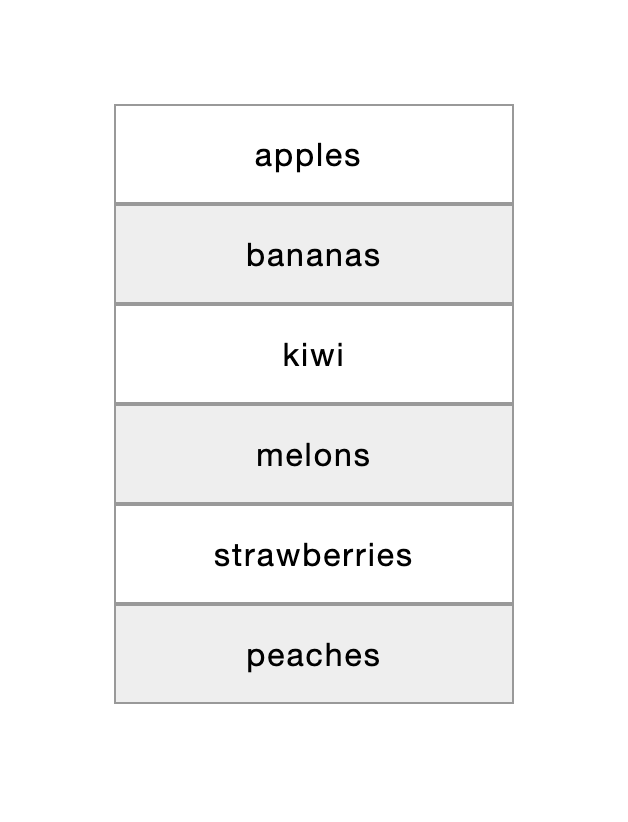Learn

# Use the pipe operator

The pipe operator "|" lets you add computed columns to a frame, which results in a new augmented frame.

To add a column to a frame, use the pipe operator "|" followed by an expression in the following format: @{column_name} = {value}.

So, to add a column "foo" with the value "bar" to a frame in cell A1, we'd use this formula:

=A1 | @foo = "bar"To better understand how it works, let's take this spreadsheet data as an example:

AB
1 product
2apples
3bananas
4kiwi
5melons
6strawberries
7peaches

Let's make a frame from the data above, and then add to the frame a column "quantity" and a column "price" with random numbers:

=FRAME(A1:A7) | @quantity = RANDBETWEEN(10,50) | @price = RANDOM() * 10The resulting frame is shown below (your result may differ due to random numbers):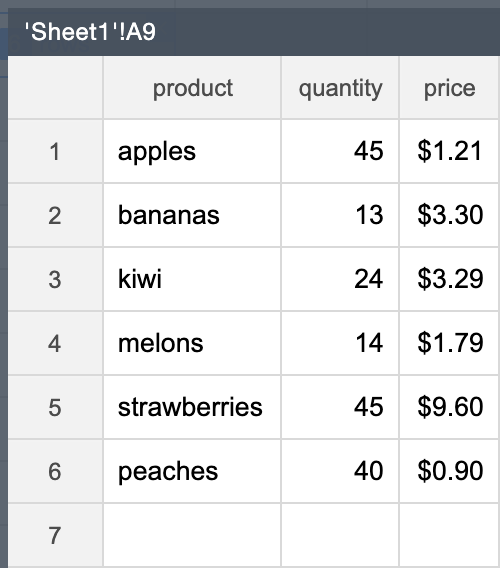Now, to add to the resulting frame a column "amount" that contains the result of multiplying the quantity by the price, use this formula:

=A9 | @amount = @quantity * @priceHere is what we get as a result: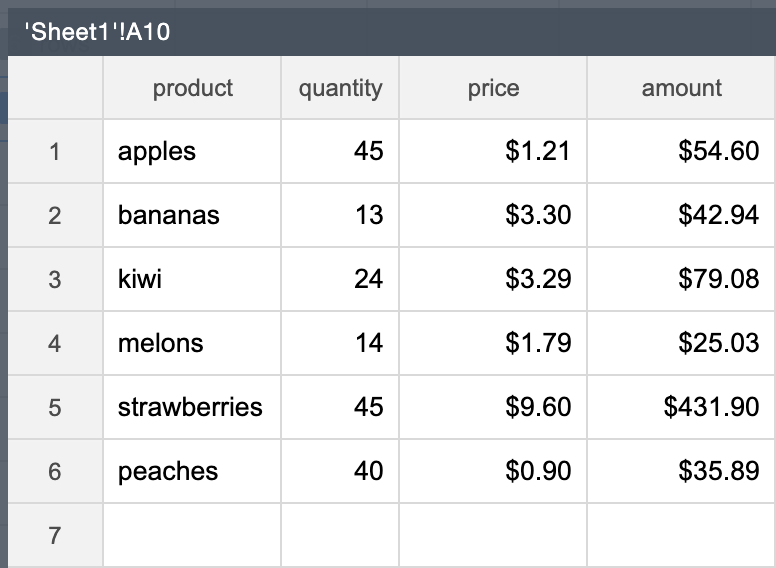Let's modify the quantity column in the frame above by deducting 10 from its values:

=A10 | @quantity = @quantity - 10The resulting frame: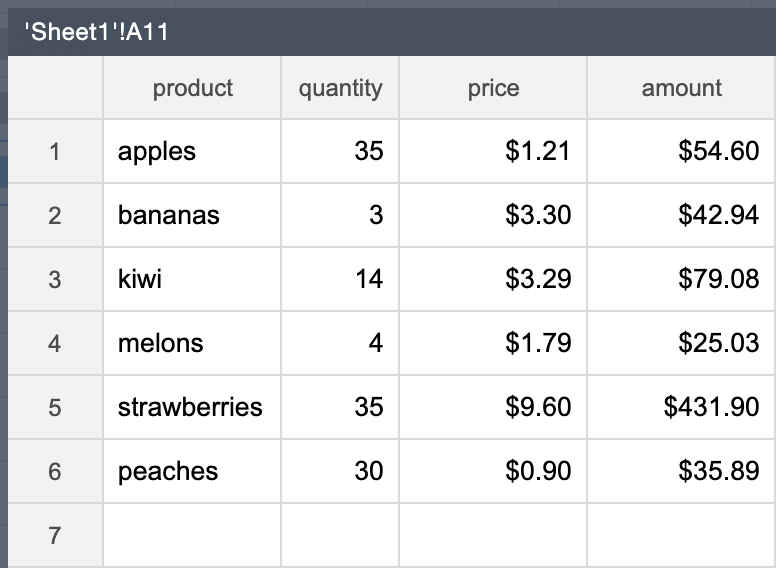## Add columns with values based on a condition

To add values based on a condition, use the IF() function in the expression.

For example, to add a column "discount" whose values are based on whether the quantity is lower than 30:

=A11 | @discount = IF(@quantity < 30, 0.2, 0.3)The resulting frame: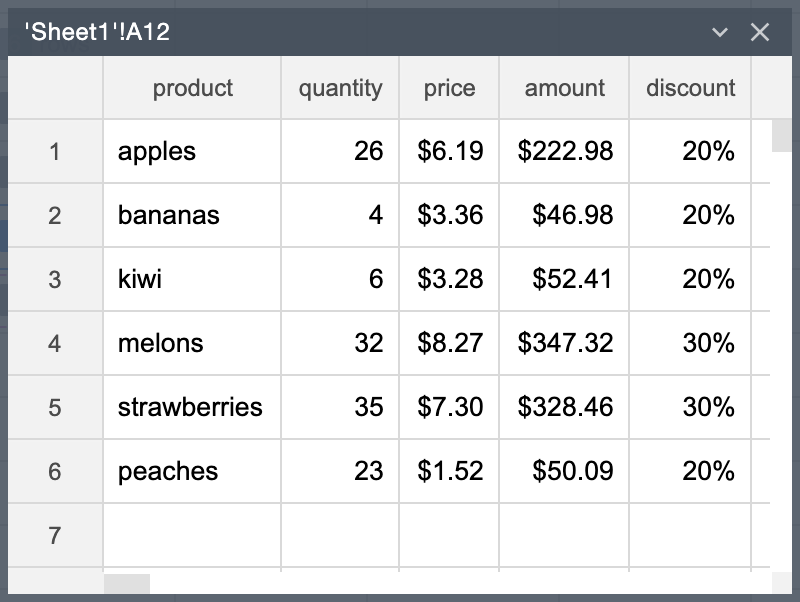Now, let's try a more convoluted example. Let's add a column "color", where the values are determined by the parity of the row number. If the row number is even, the color is gray, if the row number is odd, the color is white.

We can then use the colors to add a zebra-striping to a component in the UI.

To determine the row number, we use the special column expression @row, which returns the row number:

=A9 | @row_num = @rowThe resulting frame is shown in the figure below: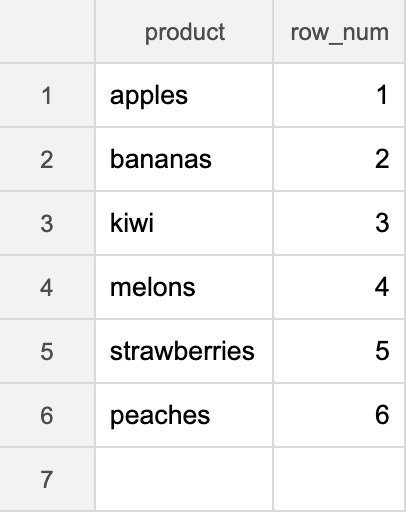To determine if the row number is even or odd, we use the MOD() function that returns the remainder of dividing the first parameter by the second parameter.

For example, the following formula returns the remainder of dividing 42 by 2 which equals 0. It means that 42 is even.

=MOD(42,2)So, given above, to add the color column to the frame, let's use this formula:

=A11 | @color = IF(MOD(@row,2), #ffffff, #eeeeee)The resulting frame: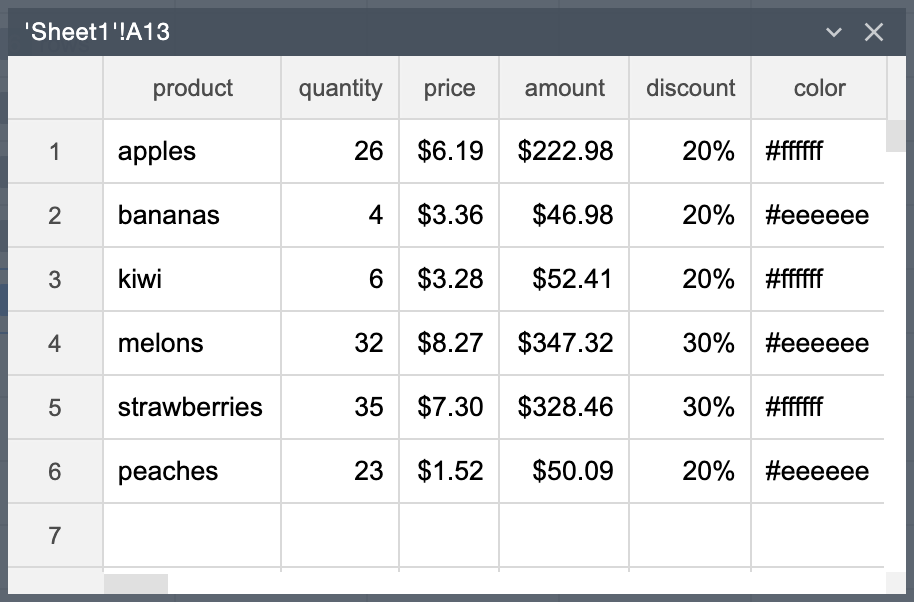Now, we can use the color to add a zebra-striping to a component on the page: